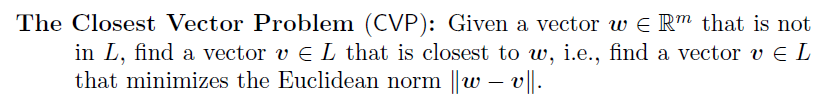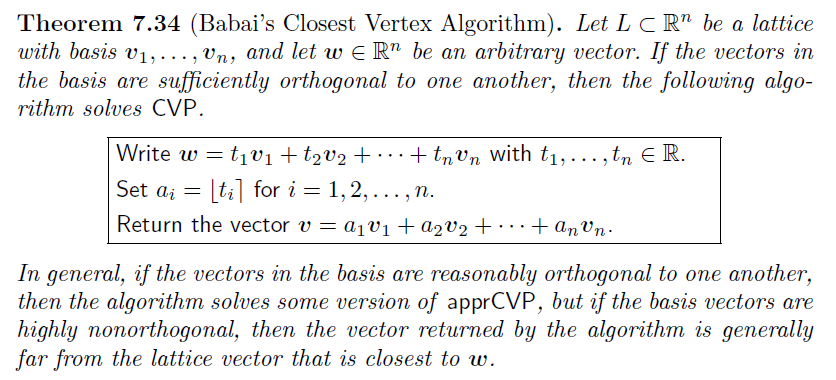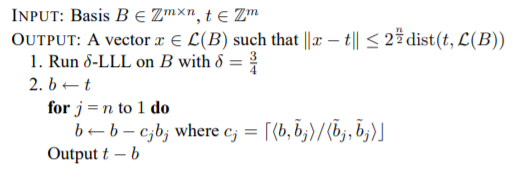# 正文

## LWE

$Ax+e=b\ (mod\ q)$

$A_{m*n}x_{n*1}+e_{m*1}=b_{m*1}\ (mod\ q)$

## CVP

CVP问题输入一个格L和一个通常不在格上的向量t，要求出在格上的离t“最近”的向量w。IMC上的定义：## 解法

$Ax+e=b\ (mod\ q)$

$Ax=b'\ (mod\ q)$

$Ax+e=b\ (mod\ q) \\ Ax+qI_mk=b-e$

$\left[ \begin{array}{c|c} A & pI_m \end{array}\right] \begin{bmatrix} x \\ k \\ \end{bmatrix} = b-e$

$\left[ \begin{array}{c|c} x & k \end{array}\right] \begin{bmatrix} A^T \\ pI_m \\ \end{bmatrix} = (b-e)^{T}$

$\left[ \begin{array}{c|c} pI_m & A \end{array}\right] \begin{bmatrix} k \\ x \\ \end{bmatrix} = b-e \\ \left[ \begin{array}{c|c} k & x \end{array}\right] \begin{bmatrix} pI_m \\ A^T \\ \end{bmatrix} = (b-e)^{T}$

## 例题

PS：写的时候参考了一下Lazzaro的代码，比较奇怪的是网上找easy matrix的wp发现代码几乎都是一样的，据说都是参考Nu1l的？震惊

CVP，LLL+Babai